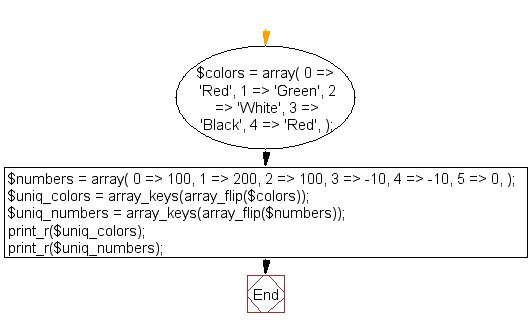﻿ PHP Array Exercise: Remove duplicate values from an array which contains only integers or strings - w3resource# PHP Array Exercises : Remove duplicate values from an array which contains only integers or strings

## PHP Array: Exercise-39 with Solution

Write a PHP program to remove duplicate values from an array which contains only strings or only integers.

Sample Solution:

PHP Code:

``````<?php
\$colors = array(
0 => 'Red',
1 => 'Green',
2 => 'White',
3 => 'Black',
4 => 'Red',
);

\$numbers = array(
0 => 100,
1 => 200,
2 => 100,
3 => -10,
4 => -10,
5 => 0,
);
\$uniq_colors = array_keys(array_flip(\$colors));
\$uniq_numbers = array_keys(array_flip(\$numbers));
print_r(\$uniq_colors);
print_r(\$uniq_numbers);
?>
```
```

Sample Output:

```Array
(
 => Red
 => Green
 => White
 => Black
)
Array
(
 => 100
 => 200
 => -10
 => 0
)
```

Flowchart:PHP Code Editor:

What is the difficulty level of this exercise?

﻿

## PHP: Tips of the Day

PHP: Extract numbers from a string

```\$str = 'In My Cart : 11 12 items';
preg_match_all('!\d+!', \$str, \$matches);
print_r(\$matches);
```